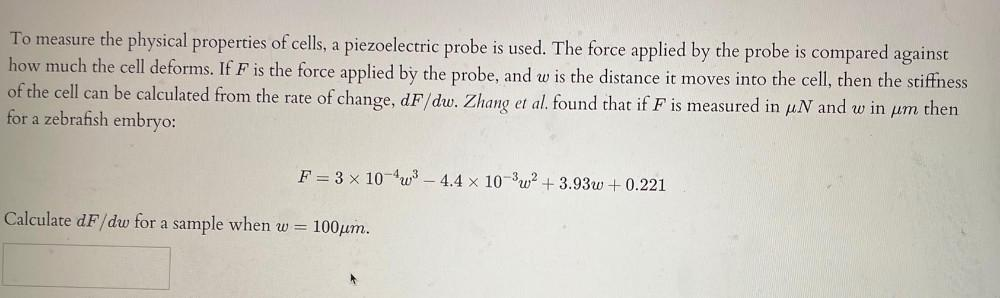Question:

# To measure the physical properties of cells, a piezoelectric probe is used. The force applied by the probe is compared against how much the cell deforms. If F is the force applied by the probe, and wTo measure the physical properties of cells, a piezoelectric probe is used. The force applied by the probe is compared against how much the cell deforms. If F is the force applied by the probe, and w is the distance it moves into the cell, then the stiffness of the cell can be calculated from the rate of change, dF/dw. Zhang et al. found that if F is measured in μN and w in μm then for a zebrafish embryo: F = 3 x 10⁻⁴ w³- 4.4 x 10⁻³w² + 3.93w+0.221 Calculate dF/dw for a sample when w= 100μm.